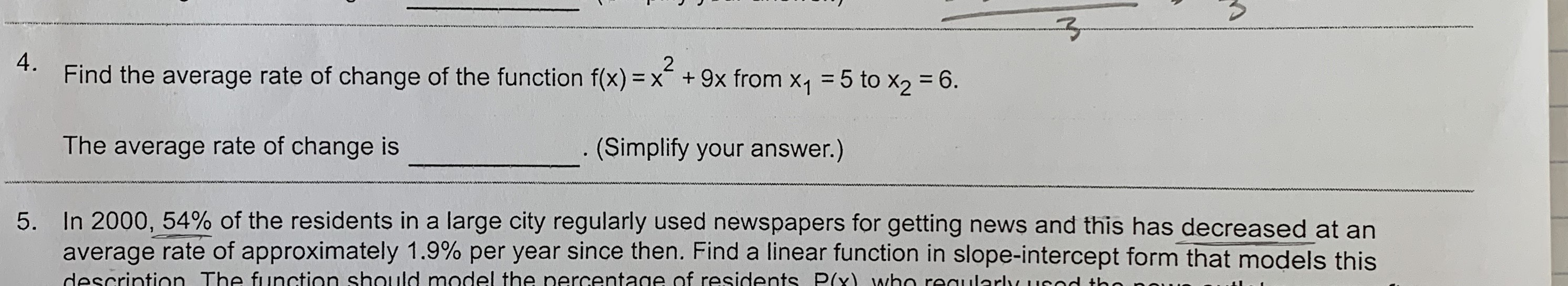4. Find the average rate of change of the function f(x) = x + 9x from X1 5 to x2 = 6.2The average rate of change is(Simplify your answer.)5. In 2000, 54% of the residents in a large city regularly used newspapers for getting news and this has decreased at anaverage rate of approximately 1.9% per year since then. Find a linear function in slope-intercept form that models thisdescrintion The function should model the nercentage of residents P(x) who reaularly usad the

Question

Can someone help me with problem four?help_outlineImage Transcriptionclose4. Find the average rate of change of the function f(x) = x + 9x from X1 5 to x2 = 6. 2 The average rate of change is (Simplify your answer.) 5. In 2000, 54% of the residents in a large city regularly used newspapers for getting news and this has decreased at an average rate of approximately 1.9% per year since then. Find a linear function in slope-intercept form that models this descrintion The function should model the nercentage of residents P(x) who reaularly usad the fullscreen
Step 1

Given,

...

Want to see the full answer?

See Solution

Want to see this answer and more?

Our solutions are written by experts, many with advanced degrees, and available 24/7

See Solution
Tagged in

Other